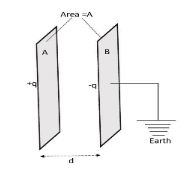QuestionAnswers

# The dielectric strength of air is $3.0\times {{10}^{8}}$V/m. A parallel plate air capacitor has area $20c{{m}^{2}}$and plate separation$\sqrt{2}mm$. Find the rms voltage of an AC source which can be safely connected to this capacitor.Verified
128.7k+ views
Hint: Dielectric strength is the breaking point of a capacitor. It can be defined as the maximum voltage or electric field required to produce a dielectric breakdown. If distance between the plates of a capacitor is d and breakdown electric field is E then breakdown voltage is given by
$V=E.d$
Root mean square voltage of an AC source having maximum value of voltage ${{V}_{0}}$ is
${{V}_{rms}}=\dfrac{{{V}_{0}}}{\sqrt{2}}$
Formula used:
$V=E.d$ and
${{V}_{rms}}=\dfrac{{{V}_{0}}}{\sqrt{2}}$

The dielectric strength or electrical strength of an insulating material is defined as the maximum electric field required to destroy dielectric or insulating property of the medium so after dielectric breakdown the charges can flow through the dielectric. It is expressed as the maximum voltage required to produce dielectric breakdown. It is expressed as Volts per unit thickness or distance between the plates.Given dielectric strength of air $E=3.0\times {{10}^{8}}$V/m.
A parallel plate air capacitor has area $20c{{m}^{2}}$ and plate separation $\sqrt{2}mm$,i.e.
$A=20c{{m}^{2}}$ and $d=\sqrt{2}mm=\sqrt{2}\times {{10}^{-3}}m$
Dielectric breakdown voltage of the capacitor is given by

$V=E.d$ where E=dielectric strength of air.
So,$V=3.0\times {{10}^{8}}{}^{V}/{}_{m}\times \sqrt{2}\times {{10}^{-3}}m=3\sqrt{2}\times {{10}^{5}}$ Volts
If an AC source is connected to this capacitor then it’s maximum voltage value should be equal to or less than $3\sqrt{2}\times {{10}^{5}}$ Volts.

The root mean square voltage of a AC source having maximum voltage V is given by

${{V}_{rms}}=\dfrac{V}{\sqrt{2}}$
Here given,$V=3\sqrt{2}\times {{10}^{5}}$Volts. So
${{V}_{rms}}=\dfrac{3\sqrt{2}\times {{10}^{5}}}{\sqrt{2}}=3\times {{10}^{5}}$Volts

Note:
For problems like this first calculate maximum value or peak value of current or potential. From the peak value you can calculate root mean square value of current and voltage. Also all units should be in S.I units. Also after dielectric breakdown there is some charge flow inside the dielectric. So the peak value of the electric field should be noted for which dielectric breakdown occurs. Charge flow will increase if you increase Electric field beyond the breakdown value.